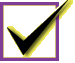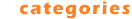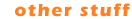Category: Education: MFileProfile Quick Pick: Acrony Our Most Popular Download and the Editor's Top Pick is a multi-player word game named Acrony. It's easy to install, quick to learn and extremely addictive. Try it!

Education : Sub-Categories
Alphabetic Education Listing
 # A B C D E F G H I J K L M N O P Q R S T U V W X Y Z

 Title - Education Alphabetic : M Education Downloads MadCalc (\$10) - MadCalc is a full featured graphing calculator application for Windows. Mathematics 1741 Magic Graph (\$19.95) - Plot and analyze graphs of mathematical functions with this powerful tool. Mathematics 622 MagicScore School (\$24.95) - Specialized notation software for music aficionados, students and schools. Other 2 MaterialWorlds Simulations (\$19.95) - Play and experiment with virtual reality simulations. Mathematics 961 Math Flight (\$11.95) - Fly High while having fun learning basic mathematics with Math Flight! Mathematics 1925 Math Function Mania (\$14.99) - Learn algebraic functions with this multi-level math game. Mathematics 748 Math Homework Maker (\$0) - Math Homework Maker,is a FREE software which can solve all your math homework Mathematics 2031 Math Worm (\$10.00) - A math puzzle where your worm navigates a maze completing arithmetic equations. Mathematics 1556 MathAid Algebra II (\$31.95) - Contain basic concepts, interactive examples, problems and customizable tests Mathematics 1901 MathAid College Algebra (\$30.95) - Java based math course includes problem-solving lessons and tests Mathematics 834 MathAid Precalculus (\$32.95) - Contain basic concepts, interactive examples, problems and customizable tests Mathematics 465 MathAid Trigonometry (\$27.95) - Java based math course includes problem-solving lessons and tests Mathematics 1826 MathCards (\$10.00) - MathCards helps students reinforce their math skills by building math equations. Mathematics 0 MatheMax Pro (\$17.95) - Practice arithmetics and counting not only for kids Kids 1733 MathMatic Junior Edition (\$0.00) - Multiplication tables driller 1 through 12. Learn your times tables with fun. Mathematics 1179 MaTris (\$17.95) - practice arithmetic skills with Tetris reward Kids 2011 MatrixCalculator (\$10) - Simple pocket calculator style matrix calculator Mathematics 1918 MB-Ruler (\$0) - tool to measure distances and angles on the desktop + screen loupe Mathematics 1708 mBasics (\$35) - Create math worksheets & assign to students for testing. Mathematics 935 MCSE Masterpack 4 (\$29.95) - Microsoft MCSE Study Guide & Questions. Core Exams 70-210,70-216 70-215, 70-217 Computer 1492 MCSE Win2K Prof. practice tests. (\$24.95) - MCSE Windows 2000 Prof. practice tests from SimulationExams.com Computer 1262 MedSpel (\$15) - Medical spelling for Microsoft Word Dictionaries 697 MemoPump (\$29) - Multilingual dictionary and words trainer MemoPump helps to increase vocabulary. Languages 2 MemoryLifter (\$0) - Multimedia flashcard for memory improvement, vocabulary & language learning Languages 1012 Micro Economy Model (\$29.90) - For studying: Price-Demand, Income, Cost and Benefit, behavior. Mathematics 1066 MOBOTSIM (\$30) - 2D simulator of mobile robots. Graphical interface, BASIC language scriptable. Science 1617 Mocha (\$0) - Sound reactive visualizations of mathematical equations. Mathematics 866 Mosquito (\$0) - Macintosh freeware for middle school mathmatics Mathematics 1334 Multi Maze Mountain 2 (\$10) - Multi Maze Mountain is a mazegame dealing with the multiplication tables. Mathematics 1647 Multilingual Translator (\$0.00) - Online translate word, sentence, passage into 10 languages ! Languages 1462 Multiple Choice Quiz Maker (\$14.95) - An easy and time-saving way to create exercises, tests and quizzes. Teaching 1140 Multiplication Table (\$6) - To learn the multiplication table!? There is nothing easier! Mathematics 1 MultyGraphiX (\$29.90) - MultyGraphix is the mathematical software designed to plot 2D and 3D functions Mathematics 1994 MusicGoals Demo (\$79.95) - ear training, sight reading, and music theory for piano, guitar, and strings Other 44 mWorksheet (\$20) - Create math worksheets for addition, subtraction, multiplication & division. Mathematics 1149 MyABCD (\$9.95) - Animated alphabet, numbers, games and music for children aged one to five Kids 1242Most Popular Editor's Choice Category Listing Audio Business Development Tools Education Games Graphics Home/Hobby Internet Miscellaneous Screen Savers UtilitiesSubmit Software Contact Us Terms of Use Other Sites Advertising

 Copyright 2004 by FileProfile.com